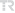## Solving of Some Random Partial Differential Equations by Using Differential Transformation Method and Laplace- Padé Method

Gümüşhane Üniversitesi Fen Bilimleri Dergisi, vol.9, no.1, pp.108-118, 2019 (Peer-Reviewed Journal)• Publication Type: Article / Article
• Volume: 9 Issue: 1
• Publication Date: 2019
• Doi Number: 10.17714/gumusfenbil.404332
• Journal Name:
• Journal Indexes: TR DİZİN (ULAKBİM)
• Page Numbers: pp.108-118
• Recep Tayyip Erdoğan University Affiliated: Yes

#### Abstract

In this study, the solutions of random partial differential equations are examined. The parameters and the initialconditions of the random component partial differential equations are investigated with Beta distribution. A fewexamples are given to illustrate the efficiency of the solutions obtained with the random Differential TransformationMethod (rDTM). Functions for the expected values and the variances of the approximate analytical solutions of therandom equations are obtained. Random Differential Transformation Method is applied to examine the solutions ofthese partial differential equations and MAPLE software is used for the finding the solutions and drawing the figures.Also the Laplace- Padé Method is used to improve the convergence of the solutions. The results for the randomcomponent partial differential equations with Beta distribution are analysed to investigate effects of this distribution onthe results. Random characteristics of the equations are compared with the results of the deterministic partial differentialequations. The efficiency of the method for the random component partial differential equations is investigated bycomparing the formulas for the expected values and variances with results from the simulations of the randomequations.